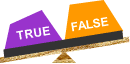# Mathematical Logic

## Mathematical logic is introduced in this unit.

Topics include sentences and statements, logical connectors, conditionals, biconditionals, equivalence and tautologies. Truth tables are constructed throughout this unit. Try our sample lessons below, or browse other instructional units.Mathematical Logic Description Negation To identify a statement as true, false or open. To list the negation of a statement in symbolic and in sentence form. To list the truth values for a given statement and its negation. Conjunction To define logical connector, compound statement, and conjunction. To list a conjunction in symbolic and in sentence form. To construct a truth table for a conjunction. Disjunction To define disjunction. To list a disjunction in symbolic and in sentence form. To construct a truth table for a disjunction. Conditional Statements To identify the hypothesis and conclusion of a conditional. To list a conditional in symbolic and in sentence form. To list the truth value of a conditional, given the value of each part. Compound Statements To evaluate sentences represented by compound statements with logical connectors. To write a compound statement in symbolic form. To construct a truth table for a compound statement to determine its truth values. Biconditional Statements To list a biconditional in symbolic and in sentence form. To identify the hypothesis and conclusion. To evaluate a sentence to determine whether or not it is biconditional. Tautologies To identify the individual parts of a compound statement. To construct a truth table for a compound statement to determine whether or not it is a tautology. Equivalence To define logical equivalence. To construct a truth table for several compound statements to determine which two are logically equivalent. To recognize that the biconditional of two equivalent statements is a tautology. Practice Exercises To complete 10 additional exercises as practice with mathematical logic. Includes interactive truth tables. To assess students' understanding of all concepts in this unit. Challenge Exercises To solve 10 additional problems that challenge students' understanding of mathematical logic. To hone students' problem-solving skills. Solutions To review complete solutions to all exercises presented in this unit. Includes the problem, step-by-step solutions, and final answer for each exercise. Learning Objectives Students Will Learn All About Negation, Conjunction, Disjunction, Conditional, Compound Statements, Biconditionals, Tautologies And Equivalence.